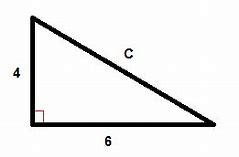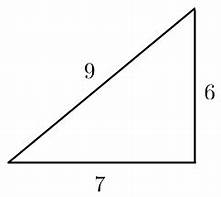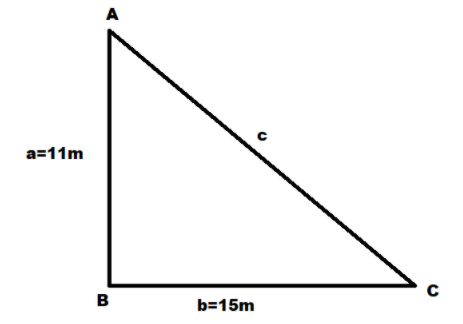Courses
Courses for Kids
Free study material
Free LIVE classes
More

# Maths Worksheet for Kids- Pythagorean Theorem Worksheet## Maths Worksheet for Kids| Pythagorean Theorem Worksheet - Download Free PDF with Solutions

The Pythagorean theorem, also known as the Pythagoras Theorem or Pythagorean Rule, is one of the most important mathematical concepts students must learn. Class KG-3 students can download the Pythagorean Theorem Worksheet to solve the questions provided there and gain a better understanding of the concepts. The theorem is very simple to understand and will not take much time.

The worksheets for the Pythagorean theorem provided by the experts at Vedantu are designed to provide a quality learning experience to the KG-3 students. They can easily download and refer to the worksheets to find the solutions to different Pythagoras Theorem questions in the best way. These questions have been hand-picked by the experts at Vedantu and provide full-fledged knowledge about the concepts.

Last updated date: 02nd Oct 2023
Total views: 21.9k
Views today: 0.21k

## Access Worksheet for Maths KG-2 Pythagorean Theorem Worksheet

The Pythagoras Theorem applied in a right-angled triangle states that the square of the side hypotenuse will be equal to the sum of the squares of the base and the perpendicular.

Let's solve some questions for further understanding -

Questions:

1. Identify the length of hypotenuse in the given triangle -Image - An Image of a Triangle

1. Pythagoras' theorem is applicable in which kind of triangles?

1. Solve for an in the given triangle -Image - An Image of a Triangle

1. Is the given figure a right-angled triangle ?Image - An Image of a Triangle

1. Find the value of the missing side.Image - An Image of a Triangle

1. Find the length of the hypotenuse.Image - An Image of a Triangle

1. Use Pythagorean Theorem to check whether the given triangle is a right-angle triangle or not.Image - An Image of a Triangle

1. Is the following Pythagorean Triplet correct?

3, 5 and 7

1. Solve for c in the given triangle -Image - An Image of a Triangle

1. In the below given right triangle, find the value of y.Image - An Image of a Triangle

1. In a right triangle, the longest side is 8 cm. One of the remaining sides is 4√3 cm long. Find the length of the other side.

1. What is the value of the missing side of the triangle?Image - An Image of a Triangle

1. ABC is a right triangle. AC is its hypotenuse. The length of side AB is 2√5. Side BC is twice side AB. Find the length of AC.

1. Find the value of x.Image - An Image of a Triangle

1. Is ∆ABC a right-angled triangle, where AB = 5cm, BC = 10cm, AC = 15cm?

1. 7.2, the length of the hypotenuse in the given triangle is 7.2.

2. Pythagoras theorem is applicable only in right-angled triangles.

3. Given, b=12 and c= 15

a2+b2=c2

a2+ 144 =225

Subtract 144 from each side to get:

144 - 144 + a2 = 225 - 144

a2 = 225 - 144 ⇒ a2 = 81

⇒ a = √81

a = 9

1. Yes, the given triangle is a right angled triangle.

2. By Pythagoras theorem, a2+b2=c2

Let b=9 c=12

144 - 81 =a2 ⇒ 63 = a2

√63 = a

Therefore, the length of the missing side is √63.

1. 10in.

2. By Pythagoras theorem, a2+b2=c2

Let a=6 b=7 c=9

36 + 49 = 81 ⇔ 9 x 9 = 81

Therefore, LHS≠RHS

Hence, the given triangle is not a right angle triangle.

1. By Pythagoras theorem, a2+b2=c2

Let a=3 b=5 c=7

9 + 25 = 34

7 × 7 = 49

Therefore, LHS≠RHS

Hence, 3, 5 and 7 are not correct Pythagorean triplets.

1. Given, a=11 b=15

By Pythagoras theorem,

a2+b2=c2

121 + 225 = c2

346 = c2

c = √346m

1. By Pythagoras theorem we get,

z2=x2+y2

Now, directly substituting the values

⇒ 169 = 25 + y2

y2 = 144 ⇒ y = 12

Hence, the value of y is 12.

1. 4cm.

2. By Pythagoras theorem, a2+b2=c2

Let a=3 b= 6

9 + 36 = c2 ⇒ 45 = c2

√45 = c

Therefore, the length of the missing side is √45cm.

1. AC = 10

2. By the pythagoras theorem, Hypotenuse2 = Base2 + Perpendicular2

x2 = 64+36 = 100

x = 10

Therefore, the value of x is 10.

1. If ∆ABC is a right-angled triangle, then AB2+BC2=AC2

AB = 5, BC =10, AC = 15

AB2 + BC2 = 25 + 100 = 125

AC2 = 225

Since, AC2AB2 + BC2

Hence, ABC is not a right-angled triangle.

### Importance of Pythagoras Theorem Worksheet for Students

Students of KG-3 will be able to gain a better understanding of the Pythagoras Theorem from these worksheets. From these questions, students will learn how to find the different sides of the right-angled triangle. The Pythagoras theorem topics have been explained in the worksheets to understand the students better. Not just that, but there are different types of sums, such as word problems, real-time applications, numerical questions, and so much more. Students will also find MCQ on Pythagoras' Theorem in these worksheets.

Students who want to complete their KG-3 Maths syllabus properly need to download these worksheets and their solutions in the first place. This will not only help them understand the examination pattern but will also improve their answering skills as well. The more they practice the questions provided here, the better it will be for their minds. Students can develop great logical thinking and cognitive abilities by solving the questions provided in these worksheets. Downloading the Pythagoras theorem sums and worksheets will help them score good marks in the examinations.

### Benefits of Pythagoras Theorem Questions and Worksheets

• For students who are in Class KG-3, it is extremely important to complete their syllabus. Thus they can take some help from the questions provided in the worksheets related to the Pythagoras theorem and understand the chapter completely.

• These worksheets have been prepared by the learned subject matter experts at Vedantu and contain all the details about the chapter. The experts have also included some special tricks and shortcuts to solve the problems easily.

• Students can download and refer to the worksheets to resolve any queries or clarify any doubts that they might have about the chapter. This will enable them to boost their preparation for the examination best.

• Students will also be able to answer questions independently after practising from these worksheets. They will understand the answering pattern with the help of the Pythagoras Theorem Worksheets PDF and can answer questions on their own.

To learn the concepts of Pythagoras' theorem best, download and practice Pythagorean Theorem Worksheet from Vedantu. These study materials are highly reliable and will help you understand the topics in the best way. Prepare well for your exams with these worksheets.

## FAQs on Maths Worksheet for Kids- Pythagorean Theorem Worksheet

Yes, the worksheet for Pythagoras theorem has been provided by Vedantu for free; hence, you don’t have to pay anything to download these worksheets.

2. How can I prepare for exams using the Pythagorean theorem worksheets?

After downloading the Pythagorean theorem worksheets from Vedantu, you can regularly practice the questions provided in the PDF files. This will enable you to gain a better understanding of the concepts.

3. Is it possible to thoroughly understand the chapter with the help of these worksheets?

Yes, you will be able to completely understand the chapter once you solve all the questions provided in the worksheet.